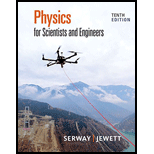Chapter 16, Problem 54APPhysics for Scientists and Enginee...

10th Edition
Raymond A. Serway + 1 other
ISBN: 9781337553278

Solutions

Chapter
SectionPhysics for Scientists and Enginee...

10th Edition
Raymond A. Serway + 1 other
ISBN: 9781337553278
Textbook Problem

Consider the following wave function in SI units: Δ P ( r , t ) = ( 25.0 r ) sin   ( 1.36 r − 2   030 t ) Explain how this wave function can apply to a wave radiating from a small source, with r being the radial distance from the center of the source to any point outside the source. Give the most detailed description of the wave that you can. Include answers to such questions as the following and give representative values for any quantities that can be evaluated. (a) Does the wave move more toward the right or the left? (b) As it moves away from the source, what happens to its amplitude? (c) Its speed? (d) Its frequency? (e) Its wavelength? (f) Its power? (g) Its intensity?

(a)

To determine

Whether the wave move toward right or the left.

Explanation

The given wave function is,

ΔP(r,t)=(25.0r)sin(1.36r2030t)        (1)

The standard form wave function for the standing wave is,

y=Asin(kxωt)        (2)

Here, A is the amplitude of the wave, k is the number of the waves, x is the position of the wave, ω is the angular frequency and t is the time period

(b)

To determine

The effect on its amplitude as it moves away from the source.

(c)

To determine

The effect on its speed as it moves away from the source.

(d)

To determine

The effect on its frequency as it moves away from the source.

(e)

To determine

The effect on its wavelength as it moves away from the source.

(f)

To determine

The effect of its power as it moves away from the source.

(e)

To determine

The effect of its intensity as it moves away from the source.

Still sussing out bartleby?

Check out a sample textbook solution.

See a sample solution

The Solution to Your Study Problems

Bartleby provides explanations to thousands of textbook problems written by our experts, many with advanced degrees!

Get Started